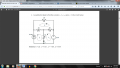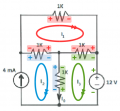# Loop Mesh Analysis to find Io,I1,I2,I3.

#### HPage5

Joined Jan 17, 2016
15
I am starting to understand this stuff a lot better now thanks to the great people in this forum who are willing to take the time to help. I have made a several attempts at this problem and end up with at least one value that is incorrect.

I know I am making an error, because in trying to solve for I1,I2, I3, I end up solving for I1, I3, and Io and I am not sure why. Maybe some one can spot my error?

Also, I dont understand how the top branch (loop I1) can have a 0 current. I have built this circuit in a simulator to double check the given values and from what I can tell they are correct.The closest I've gotten so far is this:

starting from the bottom left corner of each loop.

LI1:
I1(1k)+I1(1K)+I1(1k)=0
-->300I1=0

LI2:
-4+I2(1k)+(I2+I3)(1k)=0
-->2000I2+1000I3=0.004

LI3:
(I3+I2)(1k)+I3(1k)+12=0
-->1000I2+2000I3=-12

solving the system gives me:
I1=0ma, I2=-4ma, and I3=8ma.

I think I've got the right idea with this mesh stuff, but I'm including/excluding something somewhere that isn't correct.

Any thoughts?

#### shteii01

Joined Feb 19, 2010
4,644
I2 does not need equation. I2 is given to you by the fact that I2 is a mesh current, that mesh has a branch that only has one component, this component is an independent 4 mA current source. Therefore I2 is automatically 4 mA.

I1 equation is wrong.

I3 equation is wrong.

#### RBR1317

Joined Nov 13, 2010
706
While I never ever use mesh analysis, if I were forced to do so, then the first thing I would do is color code the mesh currents and voltage drops & polarities. To write each mesh equation I would write down each resistor in the mesh, multiply each resistor by the mesh current, subtract any opposing mesh currents (because if they are mesh currents then they are always opposing), incorporate any voltages sources in the mesh, add it all together and set it equal to zero.#### The Electrician

Joined Oct 9, 2007
2,916
I am starting to understand this stuff a lot better now thanks to the great people in this forum who are willing to take the time to help. I have made a several attempts at this problem and end up with at least one value that is incorrect.

I know I am making an error, because in trying to solve for I1,I2, I3, I end up solving for I1, I3, and Io and I am not sure why. Maybe some one can spot my error?

Also, I dont understand how the top branch (loop I1) can have a 0 current. I have built this circuit in a simulator to double check the given values and from what I can tell they are correct.

View attachment 99380

The closest I've gotten so far is this:

starting from the bottom left corner of each loop.

LI1:
I1(1k)+I1(1K)+I1(1k)=0
-->300I1=0

LI2:
-4+I2(1k)+(I2+I3)(1k)=0
-->2000I2+1000I3=0.004

LI3:
(I3+I2)(1k)+I3(1k)+12=0
-->1000I2+2000I3=-12

solving the system gives me:
I1=0ma, I2=-4ma, and I3=8ma.

I think I've got the right idea with this mesh stuff, but I'm including/excluding something somewhere that isn't correct.

Any thoughts?
In your LI1 equation, there should be terms involving I2 and I3.

In your LI2 equation, your units are wrong. The .004 term is a current, and the other terms (such as I2(1k)) are voltages.

In your LI3 equations, there should be a term involving I1.

#### WBahn

Joined Mar 31, 2012
28,185
LI2:
-4+I2(1k)+(I2+I3)(1k)=0
-->2000I2+1000I3=0.004

Any thoughts?
Yes. Always, always, ALWAYS track your units!

This should have been written

-(4 mA) +I2(1 kΩ)+(I2+I3)(1 kΩ) = 0

You can then immediately see that the first term is a current while the second and third terms, which are the products of a current and a resistance, are voltages. You can't add voltages and currents, thus you KNOW that this is wrong immediately and can save your self a bunch of time and effort coming up with an answer that is guaranteed to be wrong.

•HPage5